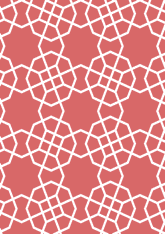# Agra Fortdata172/IND0335

## Geometry

• The symmetry group of the tiling is 4*2 (p4g).
• All the internal angles of the constituent polygons are a multiple of 45°.
• Contains two squares.
• Contains one regular 8-pointed star polygon with vertex angle of 90°.
• There are two non-regular reflective tiles.
• The tiling does not satisfy the two-colour condition.
• The tiling is edge-to-edge.
• As drawn, contains about 251 polygons.

## References

Publications referenced: